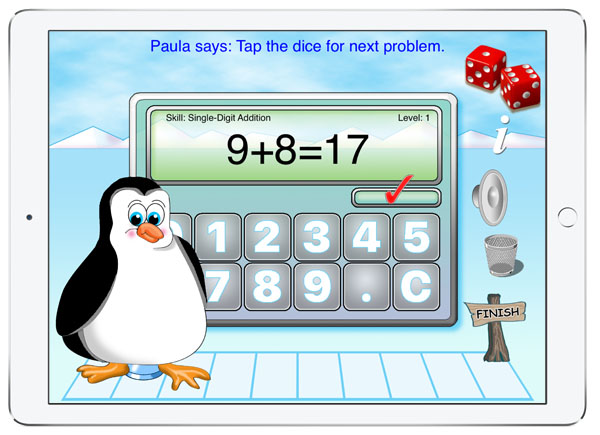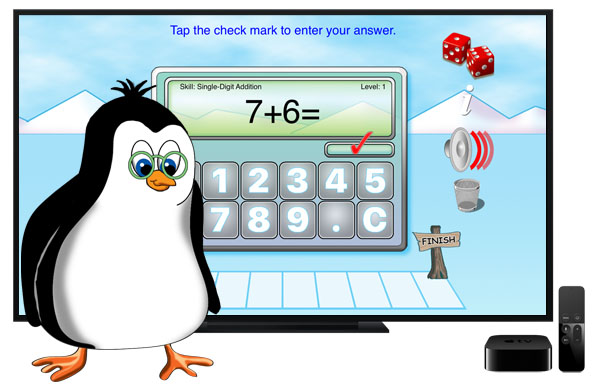Pete the Penguin's Math Game for iPad and Apple TV is a useful tool for any teacher who is interested in helping students improve their math skills.Pete the Penguin's Math Game is an interactive learning environment where student's get to practice basic math skills. One of four skills can be selected: addition, subtraction, multiplication or division. Within each skill there are five levels of difficulty. The goal is to get 10 problems correct for each skill and for each level. To begin the student selects a skill and then a marker to move along the trail. Sound effects and positive auditory feedback encourages students to do their best.Skills: Addition • Single-Digit Addition • Adding Multiples of 10 • Addition - Two-Digit • Addition - Three-Digit Plus Two-Digit • Make a 10 Addition Practice Subtraction • Single-Digit Subtraction • Subtracting 10 • Subtraction - Two-Digit Subtraction (No Regrouping) • Subtraction -Two-Digit (with Regrouping) •Three-Digit Minus a Two-Digit Number Multiplication • Multiplication Facts (0-9) • Multiplication Facts (5-12) • Multiplication by 10 or 100 • Multiplication - Two-Digit • Multiplication - Three-Digit by One-Digit Division • Division Facts (0-9) • Division Facts (5-12) • Division by 10 or 100 • Division -Two-Digit by Single-Digit • Division - Three-Digit by Single-DigitEnjoy Pete the Penguin's Math Game on your Apple TV. It is a great way to get in a little math practice before watching TV. Pete the Penguin helps kids get faster at math. It is designed to keep kids interested and focused on learning. The app can be controlled using the Siri Remote or using a Apple TV compatible game controller.